In :
%matplotlib inline


## Confidence Intervals¶

In a previous coin-flipping discussion, we discussed estimation of the underlying probability of getting a heads. There, we derived the estimator as

$$\hat{p}_n = \frac{1}{n}\sum_{i=1}^n X_i$$

Confidence intervals allow us to estimate how close we can get to the true value that we are estimating. Logically, that seems strange, doesn't it? We really don't know the exact value of what we are estimating (otherwise, why estimate it?), and yet, somehow we know how close we can get to something we admit we don't know? Ultimately, we want to make statements like the "probability of the value in a certain interval is 90%". Unfortunately, that is something we will not be able to say using our methods. Note that Bayesian estimation gets closer to this statement by using "credible intervals", but that is a story for another day. In our situation, the best we can do is say roughly the following: "if we ran the experiment multiple times, then the confidence interval would trap the true parameter 90% of the time".

Let's return to our coin-flipping example and see this in action. One way to get at a confidence interval is to use Hoeffding's inequality specialized to our Bernoulli variables as

$$\mathbb{P}(|\hat{p}_n-p|>\epsilon) \le 2 \exp(-2n \epsilon^2)$$

Now, we can form the interval $\mathbb{I}=[\hat{p}_n-\epsilon_n,\hat{p}_n+\epsilon_n]$, where $\epsilon_n$ is carefully constructed as

$$\epsilon_n = \sqrt{ \frac{1}{2 n}\log\frac{2}{\alpha}}$$

which makes the right-side of the Hoeffding inequality equal to $\alpha$. Thus, we finally have

$$\mathbb{P}(p \notin \mathbb{I}) = \mathbb{P}(|\hat{p}_n-p|>\epsilon_n) \le \alpha$$

Thus, $\mathbb{P}(p \in \mathbb{I}) \ge 1-\alpha$. As a numerical example, let's take $n=100$, $\alpha=0.05$, then plugging into everything we have gives $\epsilon_n=0.136$. So, the 95% confidence interval here is therefore

$$\mathbb{I}=[\hat{p}_n-\epsilon_n,\hat{p}_n+\epsilon_n] = [\hat{p}_n-0.136,\hat{p}_n+0.136]$$

The following code sample is a simulation to see if we can really trap the underlying parameter in our confidence interval.

In :
from scipy import stats
from scipy.stats import  bernoulli
b=bernoulli(.5) # fair coin distribution
nsamples = 100
xs = b.rvs(nsamples*200).reshape(nsamples,-1) # flip it nsamples times for 200 estimates
phat = mean(xs,axis=0) # estimated p
epsilon_n=sqrt(np.log(2/0.05)/2/nsamples) # edge of 95% confidence interval
print '--Interval trapped correct value ',np.logical_and(phat-epsilon_n<=0.5, 0.5 <= (epsilon_n +phat)).mean()*100,'% of the time'

--Interval trapped correct value  100.0 % of the time


The result in the previous cell shows that the estimator and the corresponding interval was able to trap the true value at least 95% of the time. This is how to interpret the action of confidence intervals.

However, the usual practice is to not use Hoeffding's inequality and instead use arguments around asymptotic normality. First, we need a concept of what asymptotic means.

## Types of Convergence¶

Let's consider the following sequences of random variables where $X_n = 1/2^n$ with probability $p_n$ and where $X_n=c$ with probability $1-p_n$. Then, we have $X_n \overset{P}{\to} 0$ as $p_n \rightarrow 1$. This is allowable under this notion of convergence because a diminishing amount of non-converging behavior (namely, when $X_n=c$) is possible. Note that we have said nothing about how $p_n \rightarrow 1$.

Now, let's consider the convergence in distribution case. Suppose we have $X_n \sim \mathcal{N}(0,1/n)$, which means that the variance for each of the $X_n$ gets smaller and smaller. By a quick change of variables, this means that $Z=\sqrt{n}X_n \sim \mathcal{N}(0,1)$. For $t<0$ we have the following:

$$F_n(t) = \mathbb{P}(X_n<t) =\mathbb{P}(Z<\sqrt{n}t) \rightarrow 0$$

as $n\rightarrow \infty$. Likewise, for $t>0$, we have $F_n(t) = \mathbb{P}(X_n<t) \rightarrow 1$ as $n\rightarrow \infty$.

Hence, $F_n(t) \rightarrow F(t)$ where $t\neq0$. What about $F_n(1/2)=1/2$ where $F(1/2)=1$? Convergence has failed for this point, but that does not matter for this definition of convergence because we only need convergence at the continuity points of $F(t)$.

The main thing to keep in mind about convergence in probability versus convergence in distribution is that the former is concerned about the random variables themselves whereas the latter is only about their corresponding distribution functions. This implies that for convergence in distribution, the random variables do not even have to exist in the same space or have a (function-wise) limit in the same space. This is not true for convergence in probability, which is thereby more restrictive.

Up to this point, we have been intuitively using some ideas about convergence that we now have to nail down. The first kind of convergence is convergence in probability which means the following:

$$\mathbb{P}(|X_n -X|> \epsilon) \rightarrow 0$$

as $n \rightarrow 0$. This is notationally shown as $X_n \overset{P}{\to} X$.

The second major kind of convergence is convergence in distribution where

$$\lim_{n \to \infty} F_n(t) = F(t)$$

for all $t$ for which $F$ is continuous. Bear in mind that we are talking about $F(t)$ as the probability cumulative density for $X$. This kind of convergence is usually annotated as $X_n \rightsquigarrow X$.

The third and final convergence we are interested in is quadratic mean convergence where

$$\lim_{n\rightarrow 0} \mathbb{E}(X_n-X)^2 \rightarrow 0$$

This is notationally shown as $X_n \overset{qm}{\to} X$. These forms of convergence form a hierarchy where quadratic mean convergence implies convergence in probability, which in turn, implies convergence in distribution. Except in certain special cases, this hierarchy is rigid. It turns out we need these notions in order to collect a wide range of useful results that derive from each of these categories in turn. Here is a concrete example that illustrates the different kinds of convergence.

This finally leads us to the idea of the weak law of large numbers: If $X_i$ are all independent, identically-distributed with mean $\mu=\mathbb{E}(X_1)$, then

$$\bar{X_n} \overset{P}{\to} \mu$$

It turns out that many useful estimators are asymptotically normal:

$$\frac{\hat{\theta_n}-\theta}{\texttt{se}} \rightsquigarrow \mathcal{N}(0,1)$$

## Back to confidence intervals¶

The definition of the standard error is the following:

$$\texttt{se} = \sqrt{\mathbb{V(\hat{\theta}_n)}}$$

where $\hat{\theta}_n$ is the point-estimator for the parameter $\theta$, given $n$ samples of data $X_n$. The $\mathbb{V}$ is the variance of $\hat{\theta}_n$. Likewise, the estimated standard error is $\hat{\texttt{se}}$. For example, in our coin-flipping example, the estimator was $\hat{p}=\sum X_i/n$ with corresponding variance $\mathbb{V}(\hat{p}_n)=p(1-p)/n$

we are plugging in a point estimate into a formula for the variance of $\theta$. This gives us the estimated standard error: $\hat{\texttt{se}}=\sqrt{\hat{p}(1-\hat{p})/n}$

From the result above, for our coin-flipping example, we know that $\hat{p}_n \sim \mathcal{N}(p,\widehat{\texttt{se}}^2)$. Thus, if we want a $1-\alpha$ confidence interval, we can compute

$$\mathbb{P}(|\hat{p}_n-p| \lt \xi)\gt 1-\alpha$$

but since we know that $(\hat{p}_n-p)$ is asymptotic normal, $\mathcal{N}(0,\widehat{\texttt{se}}^2)$, we can instead compute

$$\int_{-\xi}^{\xi} \mathcal{N}(0,\widehat{\texttt{se}}^2) dx \gt 1-\alpha$$

This looks ugly to compute because we need to find $\xi$, but scipy.stats has everything we need for this.

In :
se=sqrt(phat*(1-phat)/xs.shape) # compute estimated se for all trials
rv=stats.norm(0, se) # generate random variable for trial 0
array(rv.interval(0.95))+phat # compute 95% confidence interval for that trial 0

def compute_CI(i):
return stats.norm.interval(0.95,loc=i,scale=sqrt(i*(1-i)/xs.shape))
lower,upper = compute_CI(phat)

In :
fig,ax=subplots()
fig.set_size_inches((10,3))
ax.axis(ymin=0.2,ymax=0.9,xmax=100)
ax.plot(upper,label='upper asymptotic',lw=2.)
ax.plot(lower,label='lower asymptotic',lw=2.,color='b')
ax.plot(phat,'o',color='gray',label='point estimate')
ax.plot(phat+epsilon_n,label='Hoeffding upper',color='g')
ax.plot(phat-epsilon_n,label='Hoeffding lower',color='g')
ax.set_xlabel('trial index')
ax.set_ylabel('value of estimate')
ax.legend(loc=(1,0))

Out:
<matplotlib.legend.Legend at 0xc742d90>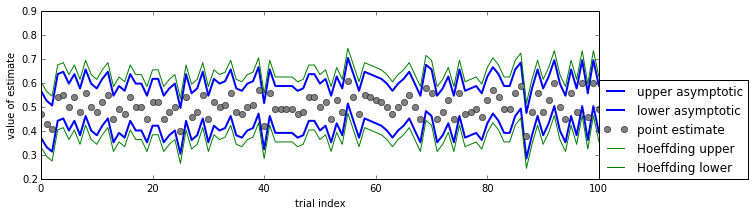The above figure shows the asymptotic confidence intervals and the Hoeffding-derived confidence intervals. As shown, the Hoeffding intervals are a bit more generous than the asymptotic estimates. However, this is only true so long as the asympotic approximation is valid. In other words, there exists some number of $n$ samples for which the asymptotic intervals may not work. So, even though they may be a bit more generous, the Hoeffding intervals do not require arguments about asymptotic convergence in order to work. In practice, nonetheless, asymptotic convergence is always in play (even if not explicitly stated).

In :
fig,ax=subplots()
xi=linspace(-3,3,100)
ax.plot(xi,stats.norm.pdf(xi))
ax.fill_between(xi[17:-17],stats.norm.pdf(xi[17:-17]),alpha=.3)
ax.text(-1,0.15,'95% probability',fontsize=18)

Out:
<matplotlib.text.Text at 0x76a8f30>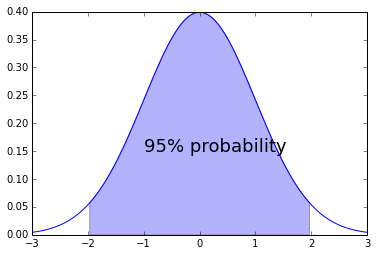## Confidence Intervals and Hypothesis testing¶

It turns out that there is a close dual relationship between hypothesis testing and the confidence intervals we have been discussing. To see this in action, consider the following hypothesis test for a normal distribution, $H_0 :\mu=\mu_0$ versus $H_1: \mu \neq \mu_0$. A reasonable test has the following rejection region:

$$\left\{ x: |\bar{x}-\mu_0| \gt z_{\alpha/2}\frac{\sigma}{\sqrt n} \right\}$$

which is the same thing as saying that the region corresponding to acceptance of $H_0$ is then, $$\bar{x} -z_{\alpha/2}\frac{\sigma}{\sqrt n} \le \mu_0 \le \bar{x} +z_{\alpha/2}\frac{\sigma}{\sqrt n}$$

Because the test has size $\alpha$, this means that $\mathbb{P}(H_0 \, \texttt{rejected}|\mu=\mu_0)=\alpha$, which is the same thing as saying the probability of false alarm. Likewise, the $\mathbb{P}(H_0 \, \texttt{accepted}|\mu=\mu_0)=1-\alpha$. Putting this all together with interval defined above means that

$$\mathbb{P}\left(\bar{x} -z_{\alpha/2}\frac{\sigma}{\sqrt n} \le \mu_0 \le \bar{x} +z_{\alpha/2}\frac{\sigma}{\sqrt n} \Big| H_0\right) =1-\alpha$$

Because this is valid for any $\mu_0$, we can drop the $H_0$ condition and say the following:

$$\mathbb{P}\left(\bar{x} -z_{\alpha/2}\frac{\sigma}{\sqrt n} \le \mu_0 \le \bar{x} +z_{\alpha/2}\frac{\sigma}{\sqrt n} \right) =1-\alpha$$

As may be obvious by now, the interval above is the $1-\alpha$ confidence interval! Thus, we have just obtained the confidence interval by inverting the acceptance region of the level $\alpha$ test. The hypothesis test fixes the parameter and then asks what sample values (i.e. the acceptance region) are consistent with that fixed value. Alternatively, the confidence interval fixes the sample value and then asks what parameter values (i.e. the confidence interval) make this sample value most plausible. Note that sometimes this inversion method results in disjoint intervals (known as confidence sets).

## Bootstrap Confidence interval¶

In :
# resample with replacement
bs=[np.random.choice(xs[:,0],size=len(xs[:,0])).mean() for i in range(100)]

In :
# use kernel density estimate to approximate empirical PDF
from scipy.stats import gaussian_kde
kbs=gaussian_kde(bs) # kernel density estimate
fig,ax=subplots()
ax.hist(bs,20,normed=True,alpha=.3);
i=linspace(.25,.7,100)
ax.plot(i,kbs.evaluate(i),lw=3.,label='kernel density\nestimate')
ax.vlines(phat,0,12,lw=4.,linestyle='--')
ax.legend()

Out:
<matplotlib.legend.Legend at 0xc92bfb0>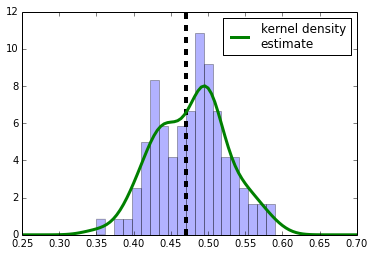In :
delta=.1
kbs.integrate_box(phat-delta,phat+delta)

Out:
0.9453581624419674
In :
from scipy.optimize import fsolve
delta=fsolve(lambda delta:0.95-kbs.integrate_box(phat-delta,phat+delta) ,0.1)

In :
fig,ax=subplots()
ax.hist(bs,20,normed=True,alpha=.3);
i=linspace(.25,.95,100)
ax.plot(i,kbs.evaluate(i),lw=3.,label='kernel density\nestimate')
ax.vlines(phat,0,12,lw=4.,linestyle='--')
ax.vlines(phat+delta,0,12,lw=4.,linestyle='--',color='gray')
ax.vlines(phat-delta,0,12,lw=4.,linestyle='--',color='gray')
ii=i[np.where(logical_and(i < phat+delta ,i>phat-delta ))]
ax.fill_between(ii,kbs.evaluate(ii),alpha=.3,color='m')
ax.legend()

Out:
<matplotlib.legend.Legend at 0xca99cf0>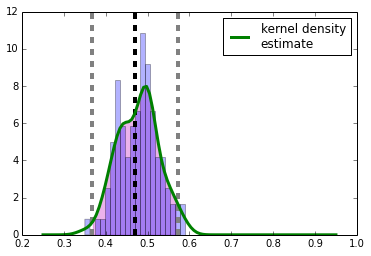In :
def compute_bootstrap_CI(x,nboot=100):
phat = x.mean()
bs=[np.random.choice(x,size=len(xs)).mean() for i in range(nboot)]
kbs=gaussian_kde(bs) # kernel density estimate
delta=fsolve(lambda delta:0.95-kbs.integrate_box(phat-delta,phat+delta) ,0.1)
return (phat-delta,phat+delta)

In :
# compute bootstrap confidence intervals
upper_b,lower_b=zip(*[ compute_bootstrap_CI(xs[:,i]) for i in range(xs.shape) ])

In :
fig,ax=subplots()
fig.set_size_inches((10,3))
ax.axis(ymin=0.2,ymax=0.9,xmax=100)
ax.plot(upper,label='upper asymptotic',lw=2.)
ax.plot(lower,label='lower asymptotic',lw=2.,color='b')
ax.plot(phat,'o',color='gray',label='point estimate')
ax.plot(phat+epsilon_n,label='Hoeffding upper',color='g')
ax.plot(phat-epsilon_n,label='Hoeffding lower',color='g')
ax.plot(upper_b,label='upper bootstrap',lw=2.,color='m')
ax.plot(lower_b,label='lower bootstrap',lw=2.,color='m')
ax.set_xlabel('trial index')
ax.set_ylabel('value of estimate')
ax.legend(loc=(1,0))

Out:
<matplotlib.legend.Legend at 0xcd83450>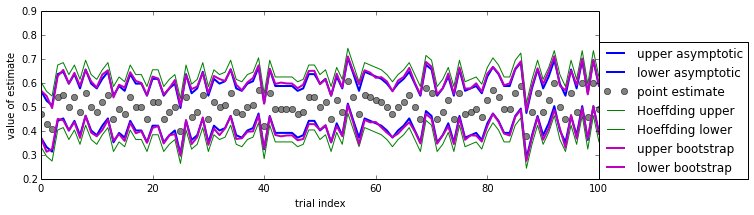## Medical Example: One-sided vs Two-sided¶

In :
# example from Good's "Common Errors in Statistics" book p.19 (which is, ironically, wrong)
from pandas import DataFrame
df=DataFrame(index=('male','female'))
df['survived']=(9,4)
df['died']=(1,10)
df

Out:
survived died
male 9 1
female 4 10

The above data shows cancer survival rates at a particular hospital. How can we determine whether or not gender has anything to do with survival? For a hypothesis testing process, we could define $H_0$ as the hypothesis that there is no gender difference in survival rates. This is actually kind of tricky to simulate, but we can get at some of the ideas below.

In :
import itertools as it
import combinatorics # from pypi.org
from collections import Counter

patients = ['M']*10 + ['F']*14
sample= np.random.permutation(patients)[:13] # use the first 13 slots for survivors
print sample
print Counter(sample)

['F' 'F' 'F' 'F' 'M' 'M' 'F' 'M' 'F' 'F' 'M' 'F' 'F']
Counter({'F': 9, 'M': 4})


The code above shows how to use a random permutation and the first 13 slots in the list to indicate the survivors in that permutation. Then, all you have to do is count the number of males and females in the first 13 slots. To get all ppossible permutations counted and divided this way, we can use a third-party combinatorics module as shown in the code below.

In :
foo = lambda i: len(set(range(10)) & set( i )) # count males in first group of 10 males total
o=[foo(i) for i in combinatorics.labeled_balls_in_unlabeled_boxes(24,[13,11]) ]

In :
hist(o,10,align='left')
title('Surviving males under $H_0$')
xlabel('number of surviving males')
axis(xmin=0);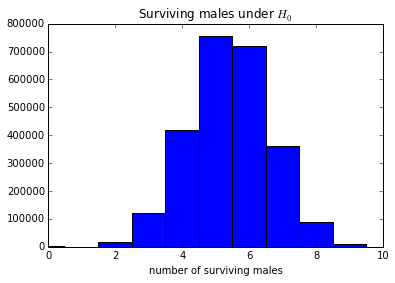In :
# probability of observing 9 male survivors under H_0 is the following
co=Counter(o)
print 'p-value= ',(co+co)/sum(co.values())
print 'p-value= ',(co+co+co+co+co)/sum(co.values())

p-value=  0.00415601023018
p-value=  0.0110883025979

In :
#using one-way chi-squared proportion test
from scipy import stats
print stats.chisquare( [9,4],[6.5,6.5])
print stats.fisher_exact(df.values)

(1.9230769230769231, 0.16551785869746993)
(22.5, 0.0045261811818548964)

In [ ]: